Functions and CALL Routines

# RAND Function

Generates random numbers from a distribution that you specify.
 Category: Random Number

## Syntax

 RAND (dist, parm-1,...,parm-k)

### Arguments

dist

is a character constant, variable, or expression that identifies the distribution. Valid distributions are as follows:

Distribution Argument
Bernoulli BERNOULLI
Beta BETA
Binomial BINOMIAL
Cauchy CAUCHY
Chi-Square CHISQUARE
Erlang ERLANG
Exponential EXPONENTIAL
F F
Gamma GAMMA
Geometric GEOMETRIC
Hypergeometric HYPERGEOMETRIC
Lognormal LOGNORMAL
Negative binomial NEGBINOMIAL
Normal NORMAL|GAUSSIAN
Poisson POISSON
T T
Tabled TABLE
Triangular TRIANGLE
Uniform UNIFORM
Weibull WEIBULL

Note:   Except for T and F, you can minimally identify any distribution by its first four characters.parm-1,...,parm-k

are shape, location, or scale parameters appropriate for the specific distribution.

 See: Details for complete information about these parameters

### Generating Random Numbers

The RAND function generates random numbers from various continuous and discrete distributions. Wherever possible, the simplest form of the distribution is used.

The RAND function uses the Mersenne-Twister random number generator (RNG) that was developed by Matsumoto and Nishimura (1998). The random number generator has a very long period (219937 - 1) and very good statistical properties. The period is a Mersenne prime, which contributes to the naming of the RNG. The algorithm is a twisted generalized feedback shift register (TGFSR) that explains the latter part of the name. The TGFSR gives the RNG a very high order of equidistribution (623-dimensional with 32-bit accuracy), which means that there is a very small correlation between successive vectors of 623 pseudo-random numbers.

The RAND function is started with a single seed. However, the state of the process cannot be captured by a single seed. You cannot stop and restart the generator from its stopping point.

### Reproducing a Random Number Stream

If you want to create reproducible streams of random numbers, then use the CALL STREAMINIT routine to specify a seed value for random number generation. Use the CALL STREAMINIT routine once per DATA step before any invocation of the RAND function. If you omit the call to the CALL STREAMINIT routine (or if you specify a non-positive seed value in the CALL STREAMINIT routine), then RAND uses a call to the system clock to seed itself. For more information, see CALL STREAMINIT Creating a Reproducible Stream of Random Numbers.

## Syntax

 x = RAND('BERNOULLI',p)

where

x

is an observation from the distribution with the following probability density function:
Range: x = 0, 1
p

is a numeric probability of success.

 Range: 0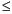p1

## Syntax

 x = RAND('BETA',a,b)

where

x

is an observation from the distribution with the following probability density function:
Range: 0 < x < 1
a

is a numeric shape parameter.

 Range: a > 0
b

is a numeric shape parameter.

 Range: b > 0

## Syntax

 x = RAND('BINOMIAL',p,n)

where

x

is an integer observation from the distribution with the following probability density function:
Range: x = 0, 1, ..., n
p

is a numeric probability of success.

 Range: 0p1
n

is an integer parameter that counts the number of independent Bernoulli trials.

 Range: n = 1, 2, ...

## Syntax

 x = RAND('CAUCHY')

where

x

is an observation from the distribution with the following probability density function:
Range: -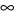< x <## Syntax

 x = RAND('CHISQUARE',df)

where

x

is an observation from the distribution with the following probability density function:
Range: x > 0
df

is a numeric degrees of freedom parameter.

 Range: df > 0

## Syntax

 x = RAND('ERLANG',a)

where

x

is an observation from the distribution with the following probability density function:
Range: x > 0
a

is an integer numeric shape parameter.

 Range: a = 1, 2, ...

## Syntax

 x = RAND('EXPONENTIAL')

where

x

is an observation from the distribution with the following probability density function: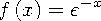Range: x > 0

## Syntax

 x = RAND('F',ndf, ddf)

where

x

is an observation from the distribution with the following probability density function:
Range: x > 0
ndf

is a numeric numerator degrees of freedom parameter.

 Range: ndf > 0
ddf

is a numeric denominator degrees of freedom parameter.

 Range: ddf > 0

## Syntax

 x = RAND('GAMMA',a)

where

x

is an observation from the distribution with the following probability density function:Range: x > 0
a

is a numeric shape parameter.

 Range: a > 0

## Syntax

 x = RAND('GEOMETRIC',p)

where

x

is an integer count that denotes the number of trials that are needed to obtain one success. X is an integer observation from the distribution with the following probability density function:
Range: x = 1, 2, ...
p

is a numeric probability of success.

 Range: 0 < p1

## Syntax

 x = RAND('HYPER',N,R,n)

where

x

is an integer observation from the distribution with the following probability density function:
Range: x = max(0, (n - (N - R))), ..., min(n, R)
N

is an integer population size parameter.

 Range: N = 1, 2, ...
R

is an integer number of items in the category of interest.

 Range: R = 0, 1, ..., N
n

is an integer sample size parameter.

 Range: n = 1, 2, ..., N

The hypergeometric distribution is a mathematical formalization of an experiment in which you draw n balls from an urn that contains N balls, R of which are red. The hypergeometric distribution is the distribution of the number of red balls in the sample of n.

## Syntax

 x = RAND('LOGNORMAL')

where

x

is an observation from the distribution with the following probability density function:
Range: x > 0

## Syntax

 x = RAND('NEGBINOMIAL',p,k)

where

x

is an integer observation from the distribution with the following probability density function:
Range: x = 0, 1, ...
k

is an integer parameter that is the number of successes. However, non-integer k values are allowed as well.

 Range: k = 1, 2, ...
p

is a numeric probability of success.

 Range: 0 < p1

The negative binomial distribution is the distribution of the number of failures before k successes occur in sequential independent trials, all with the same probability of success, p.

## Syntax

 x = RAND('NORMAL',<,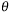,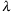>)

where

x

is an observation from the normal distribution with a mean ofand a standard deviation of, that has the following probability density function:
Range: -< x <is the mean parameter.

 Default: 0is the standard deviation parameter.

 Default: 1 Range:> 0

## Syntax

 x = RAND('POISSON',m)

where

x

is an integer observation from the distribution with the following probability density function:
Range: x = 0, 1, ...
m

is a numeric mean parameter.

 Range: m > 0

## Syntax

 x = RAND('T',df)

where

x

is an observation from the distribution with the following probability density function:
Range: -< x <df

is a numeric degrees of freedom parameter.

 Range: df > 0

## Syntax

 x = RAND('TABLE',p1,p2, ...)

where

x

is an integer observation from one of the following distributions:

If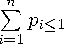, then x is an observation from this probability density function:
If for some index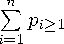, then x is an observation from this probability density function:
p1, p2, ...

are numeric probability values.

 Range: 0p1, p2, ...1 Restriction: The maximum number of probability parameters depends on your operating environment, but the maximum number of parameters is at least 32,767.

The tabled distribution takes on the values 1, 2, ..., n with specified probabilities.

Note:   By using the FORMAT statement, you can map the set {1, 2, ..., n} to any set of n or fewer elements.## Syntax

 x = RAND('TRIANGLE',h)

where

x

is an observation from the distribution with the following probability density function:
where 0h1.
 Range: 0x1

Note:   The distribution can be easily shifted and scaled.h

is the horizontal location of the peak of the triangle.

 Range: 0h1

## Syntax

 x = RAND('UNIFORM')

where

x

is an observation from the distribution with the following probability density function: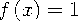Range: 0 < x < 1

The uniform random number generator that the RAND function uses is the Mersenne-Twister (Matsumoto and Nishimura 1998). This generator has a period of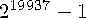and 623-dimensional equidistribution up to 32-bit accuracy. This algorithm underlies the generators for the other available distributions in the RAND function.

## Syntax

 x = RAND('WEIBULL',a,b)

where

x

is an observation from the distribution with the following probability density function:
Range: x0
a

is a numeric shape parameter.

 Range: a > 0
b

is a numeric scale parameter.

 Range: b > 0

SAS Statements Results
`x=rand('BERN',.75);`
`0`
`x=rand('BETA',3,0.1);`
`.99920`
`x=rand('BINOM',10,0.75);`
`10`
`x=rand('CAUCHY');`
`-1.41525`
`x=rand('CHISQ',22);`
`25.8526`
`x=rand('ERLANG', 7);`
`7.67039`
`x=rand('EXPO');`
`1.48847`
`x=rand('F',12,322);`
`1.99647`
`x=rand('GAMMA',7.25);`
`6.59588`
`x=rand('GEOM',0.02);`
`43`
`x=rand('HYPER',10,3,5);`
`1`
`x=rand('LOGN');`
`0.66522`
`x=rand('NEGB',0.8,5);`
`33`
`x=rand('NORMAL');`
`1.03507`
`x=rand('POISSON',6.1);`
`6`
`x=rand('T',4);`
`2.44646`
`x=rand('TABLE',.2,.5);`
`2`
`x=rand('TRIANGLE',0.7);`
`.63811`
`x=rand('UNIFORM');`
`.96234`
`x=rand('WEIB',0.25,2.1);`
`6.55778`

 CALL Routine:

 Fishman, G. S. 1996. Monte Carlo: Concepts, Algorithms, and Applications. New York: Springer-Verlag. Fushimi, M., and S. Tezuka. 1983. "The k-Distribution of Generalized Feedback Shift Register Pseudorandom Numbers." Communications of the ACM 26: 516-523. Gentle, J. E. 1998. Random Number Generation and Monte Carlo Methods. New York: Springer-Verlag. Lewis, T. G., and W. H. Payne. 1973. "Generalized Feedback Shift Register Pseudorandom Number Algorithm." Journal of the ACM 20: 456-468. Matsumoto, M., and Y. Kurita. 1992. "Twisted GFSR Generators." ACM Transactions on Modeling and Computer Simulation 2: 179-194. Matsumoto, M., and Y. Kurita. 1994. "Twisted GFSR Generators II." ACM Transactions on Modeling and Computer Simulation 4: 254-266. Matsumoto, M., and T. Nishimura. 1998. "Mersenne Twister: A 623-Dimensionally Equidistributed Uniform Pseudo-Random Number Generator." ACM Transactions on Modeling and Computer Simulation 8: 3-30. Ripley, B. D. 1987. Stochastic Simulation. New York: Wiley. Robert, C. P., and G. Casella. 1999. Monte Carlo Statistical Methods. New York: Springer-Verlag. Ross, S. M. 1997. Simulation. San Diego: Academic Press.Previous Page | Next Page | Top of Page